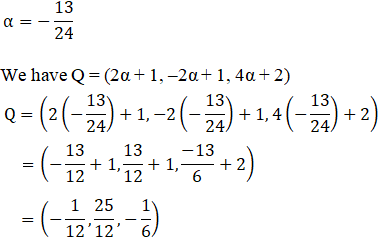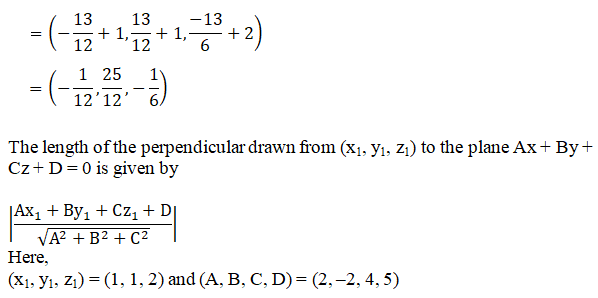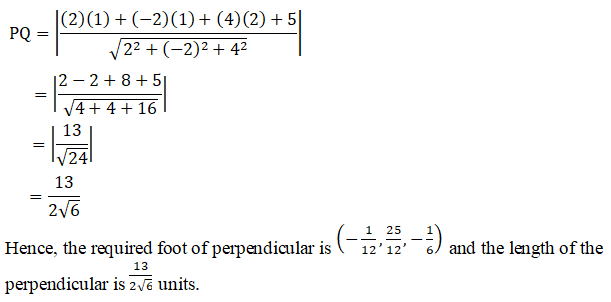# RD Sharma Solutions Class 12 The Plane Exercise 29.15

RD Sharma Solutions for Class 12 Maths Chapter 29 The Plane Exercise 29.15, is provided here for students to prepare for their exams at ease. Students can refer to RD Sharma Solutions for Class 12, which is the best reference material developed by our expert tutors, to help students gain knowledge and to have a good grip over the subject. Solving these exercises will ensure students obtain a good score in their exams. The pdf consisting of this chapter solutions which are available for free can be downloaded from the links given below.

## Download PDF of RD Sharma Solutions For Class 12 Maths Chapter 29 Exercise 15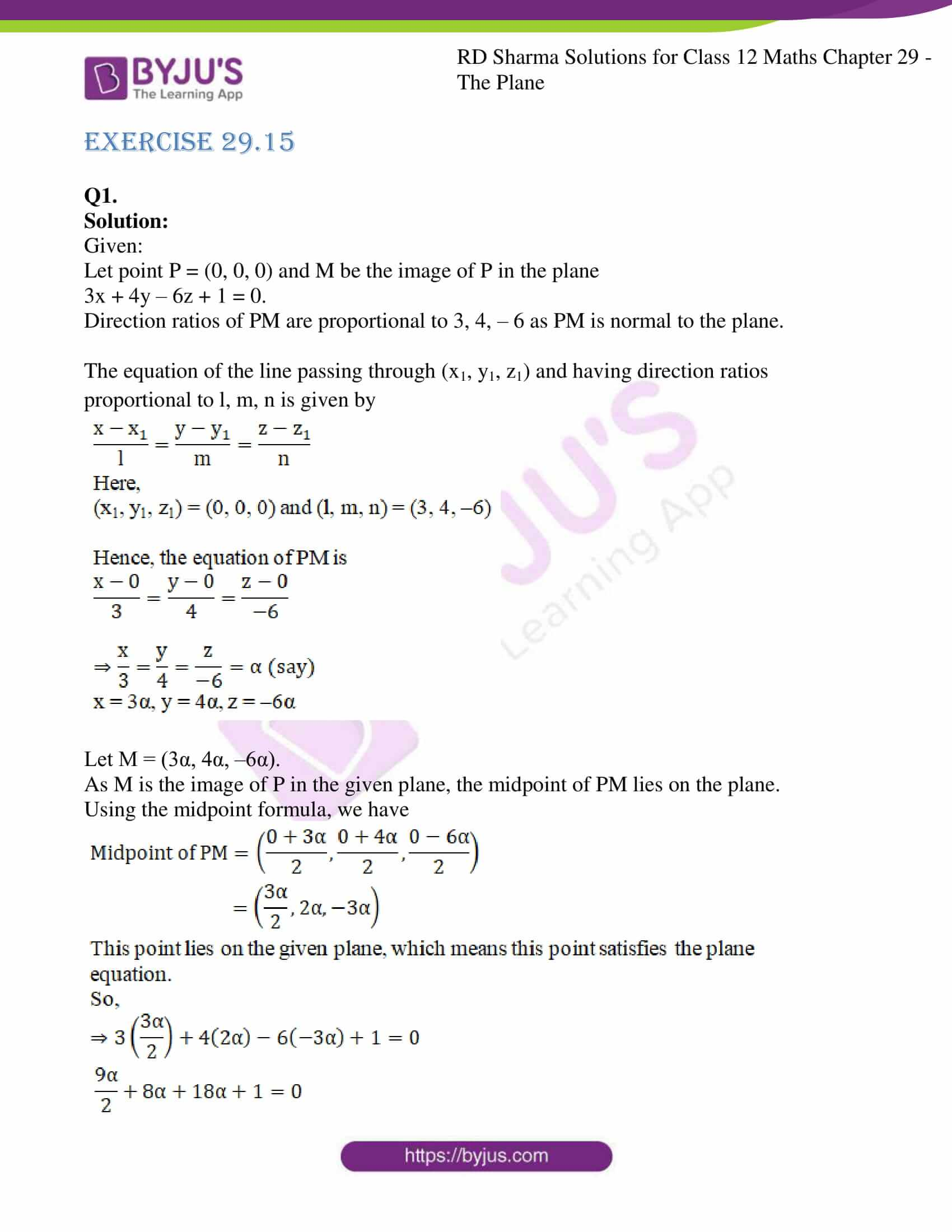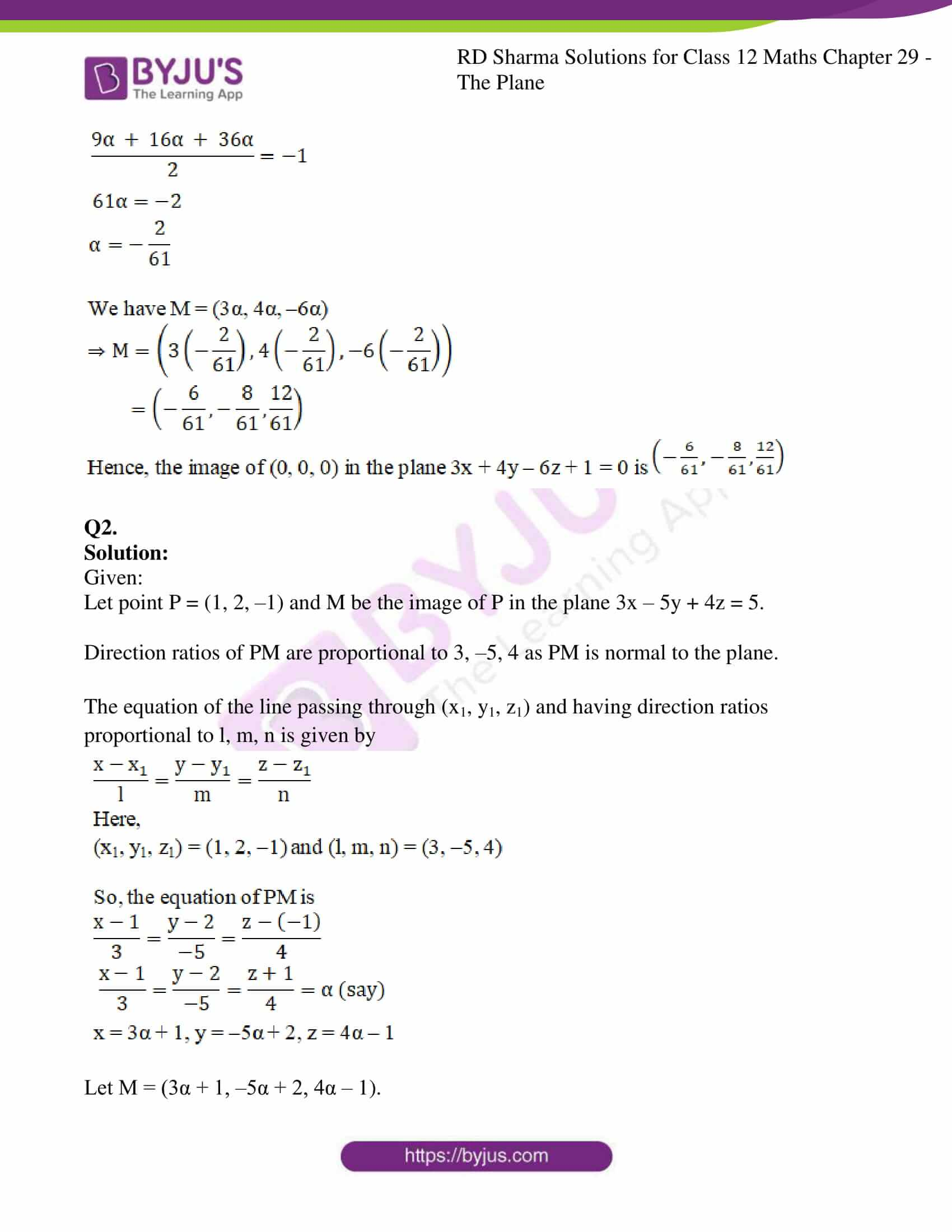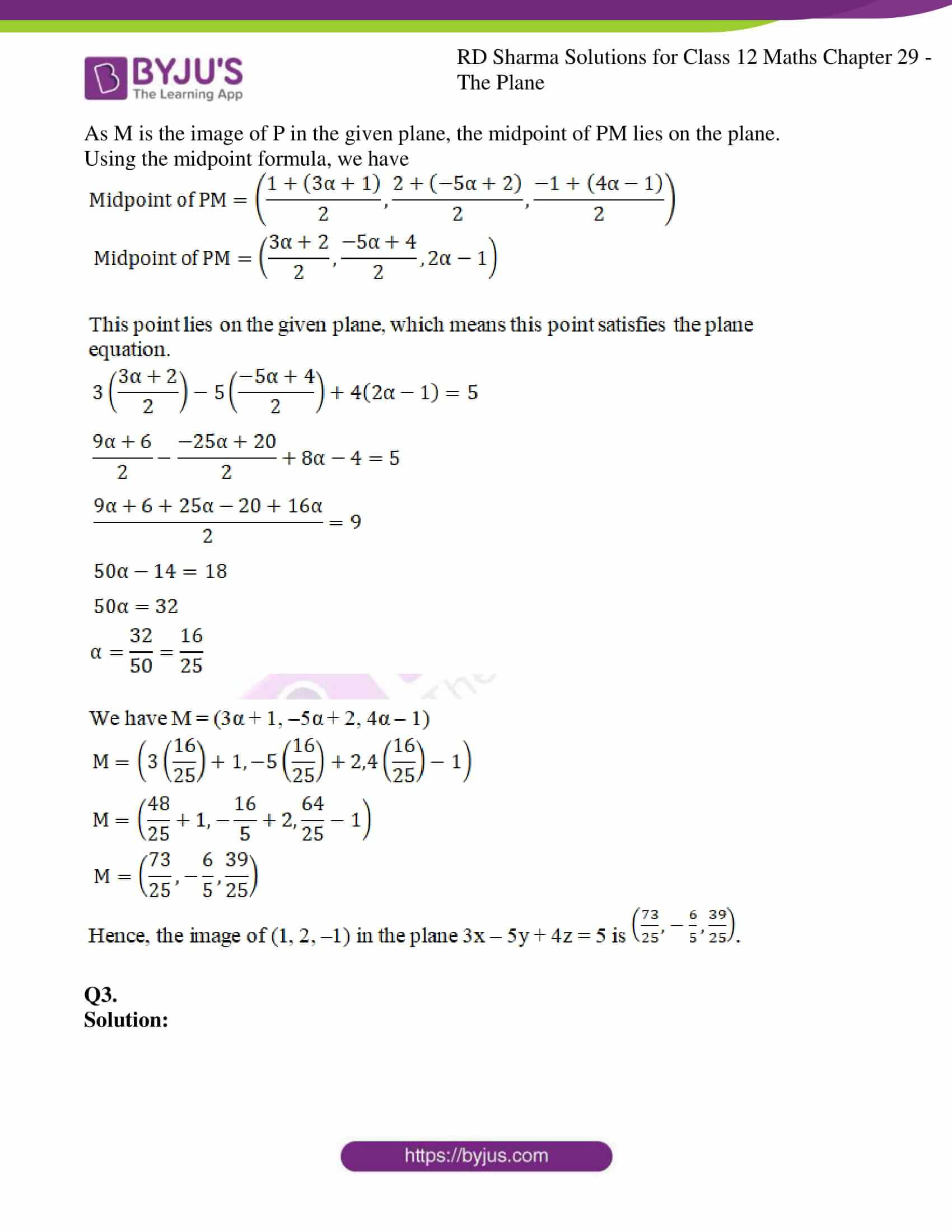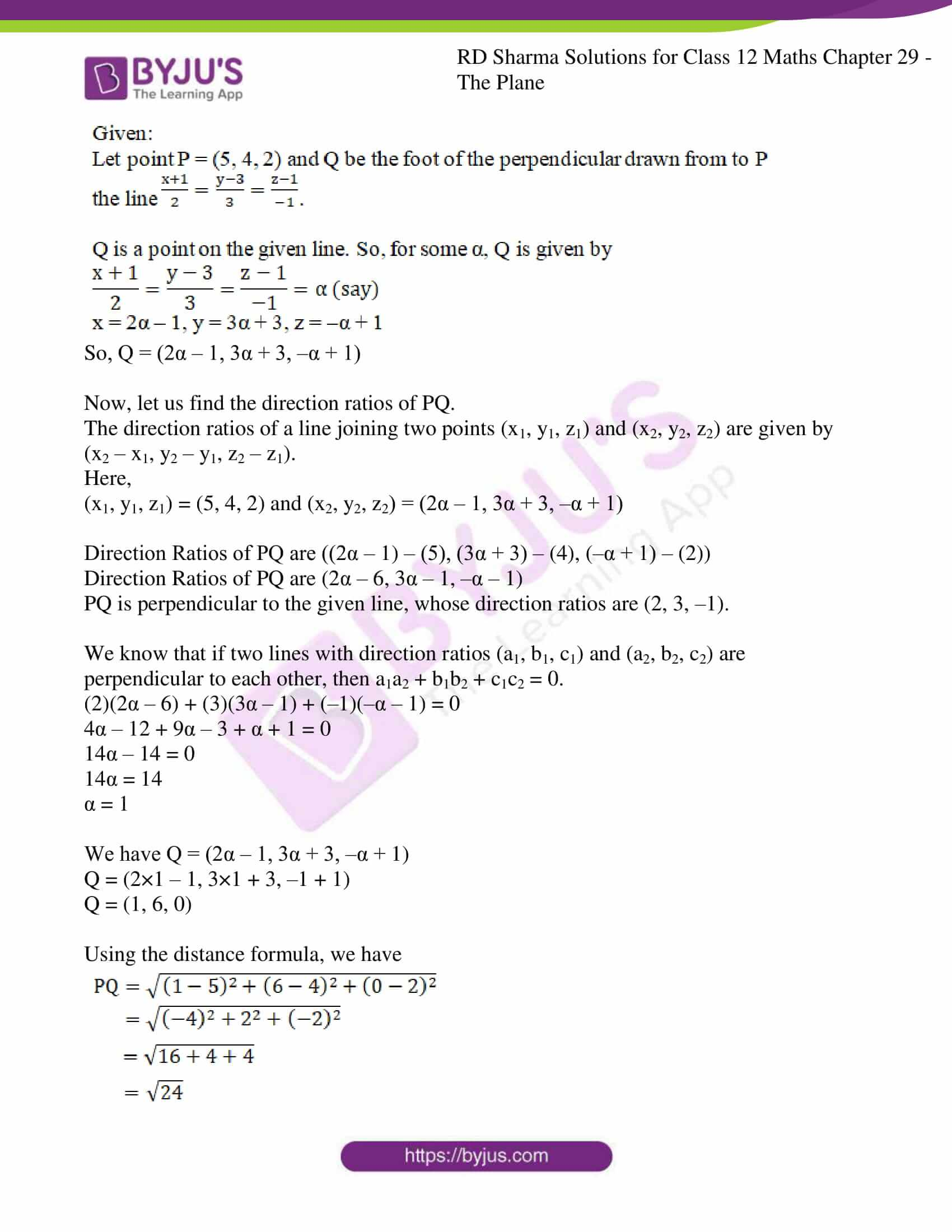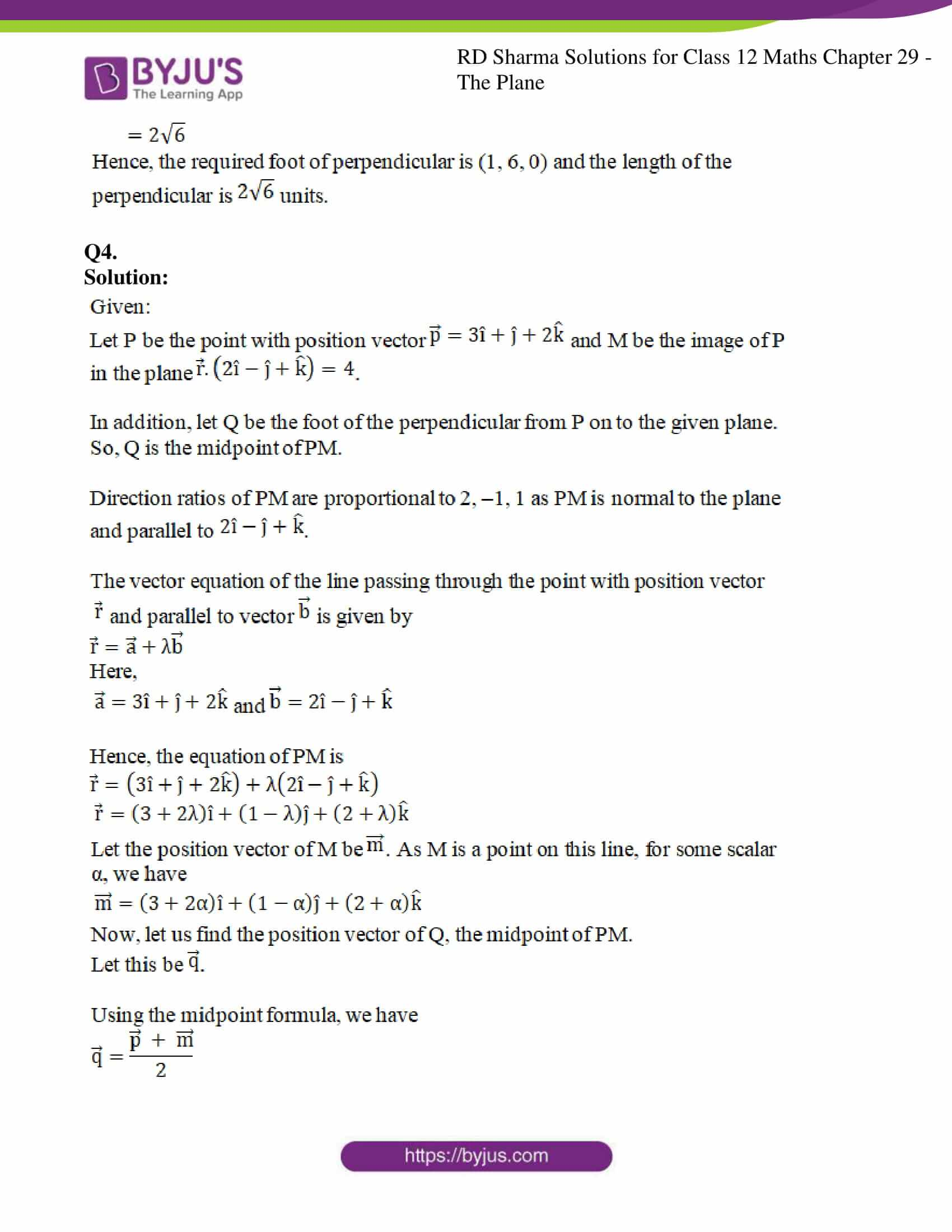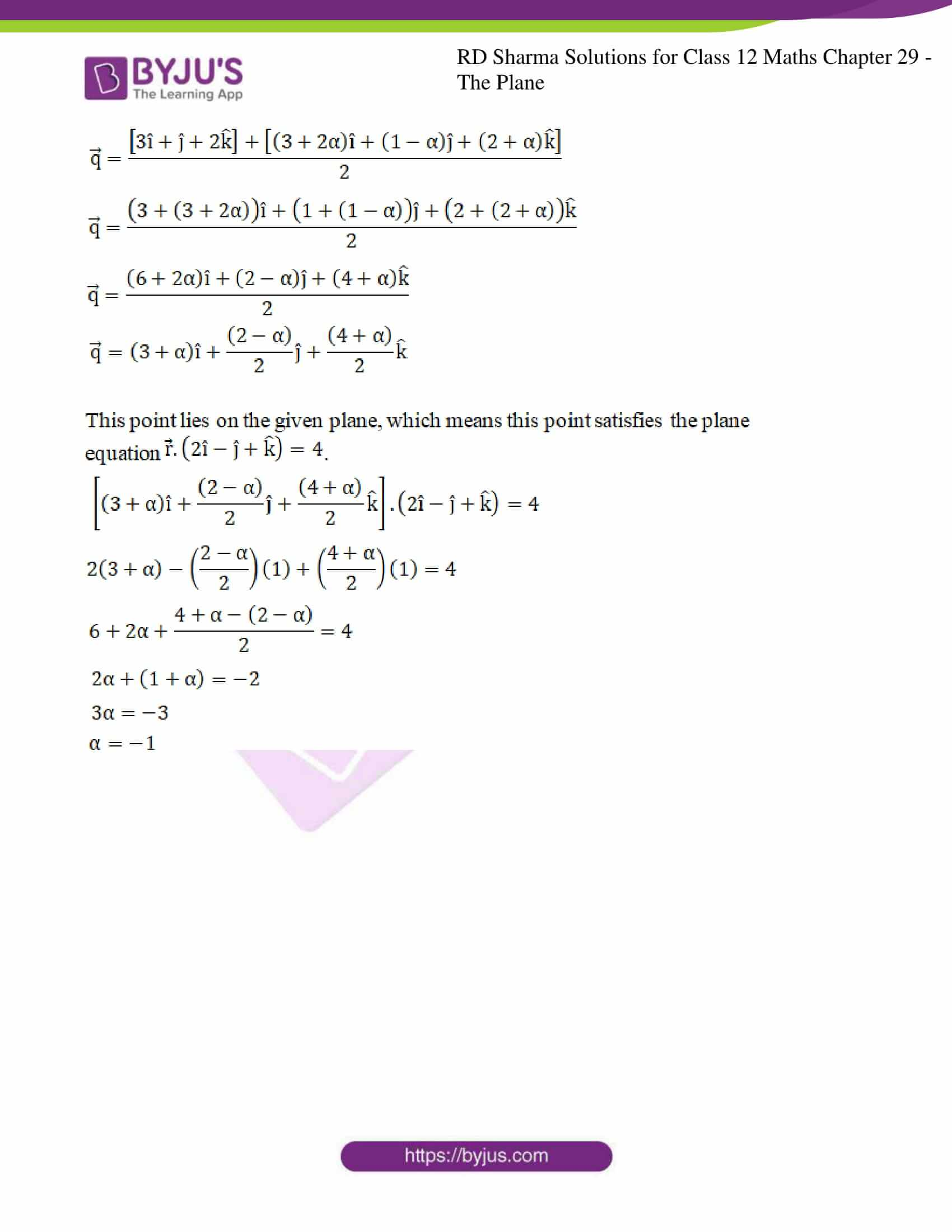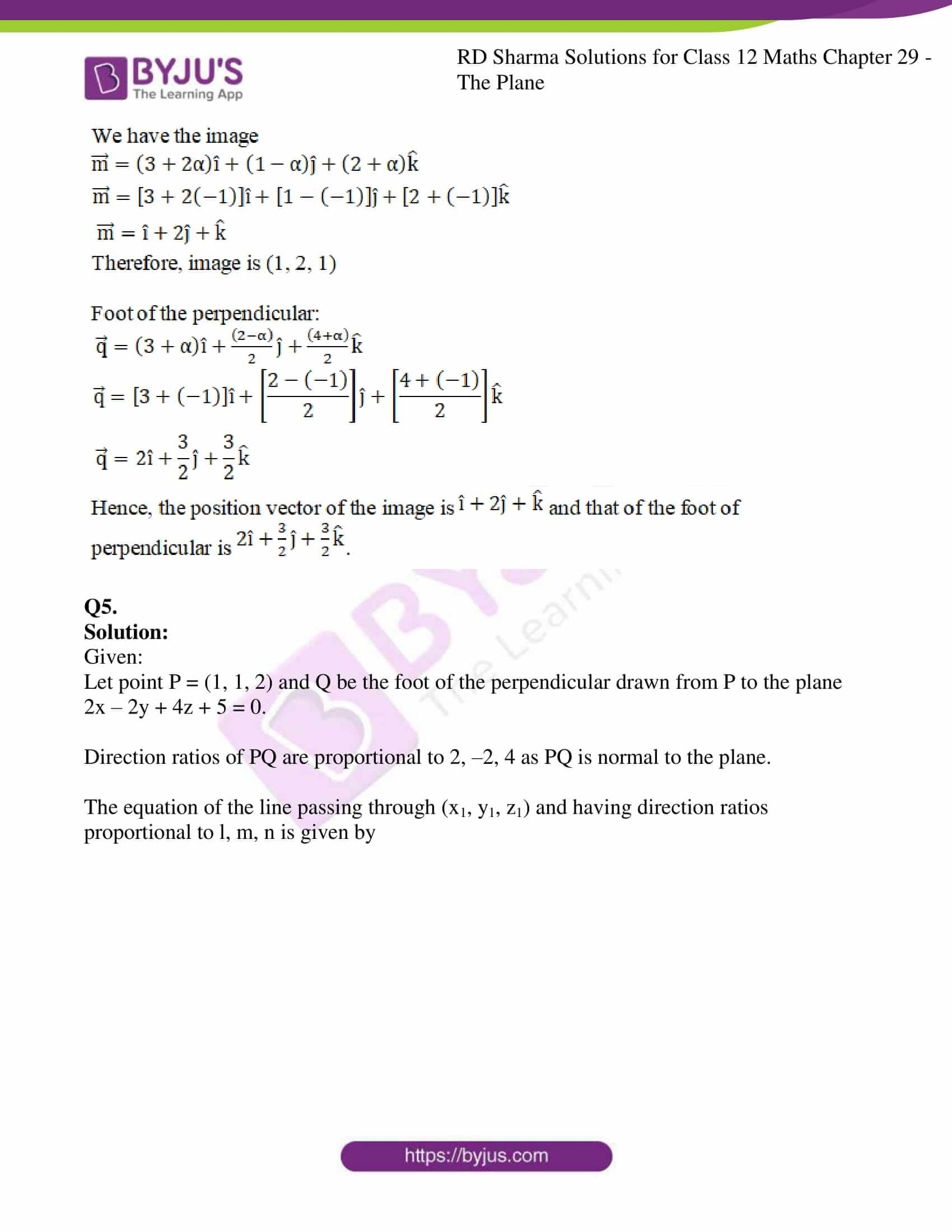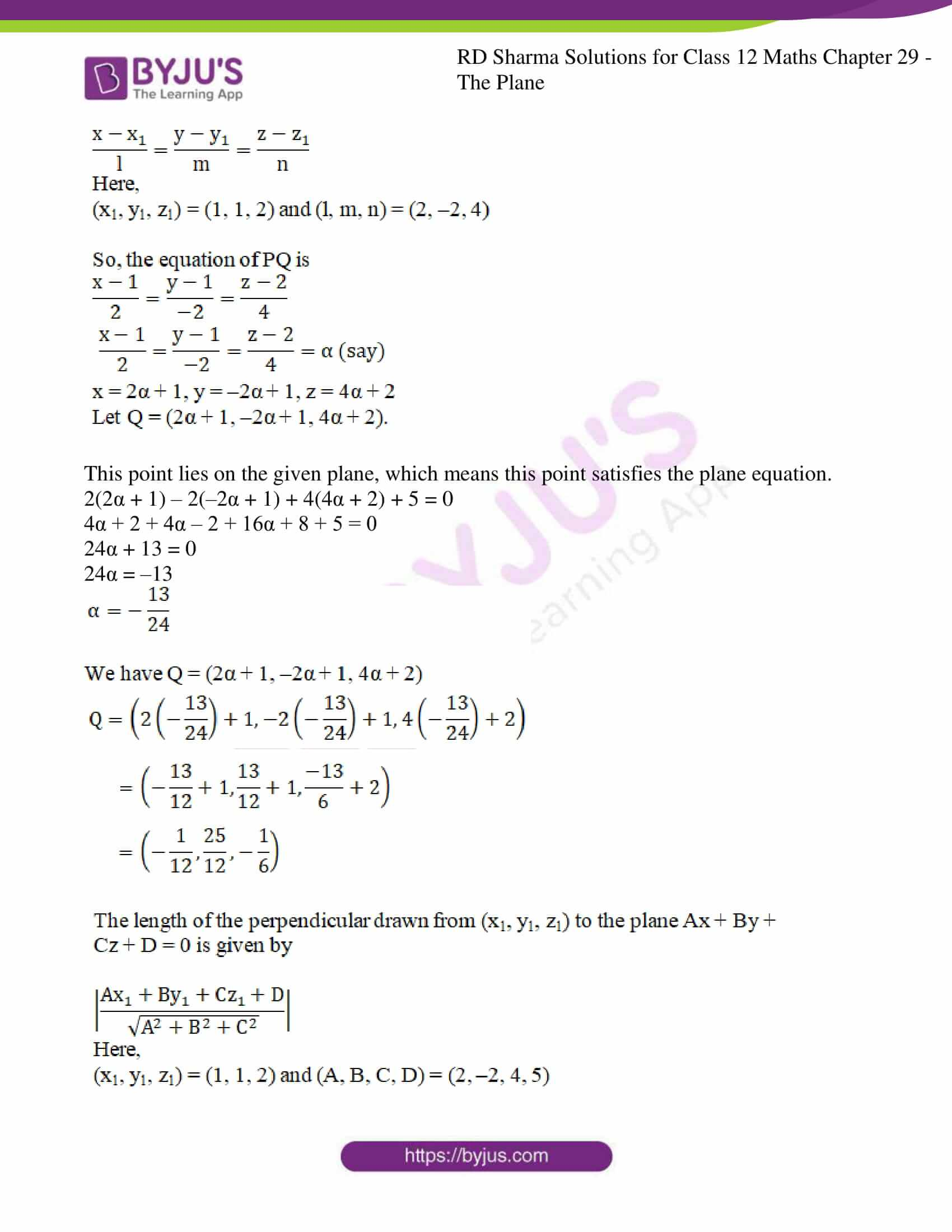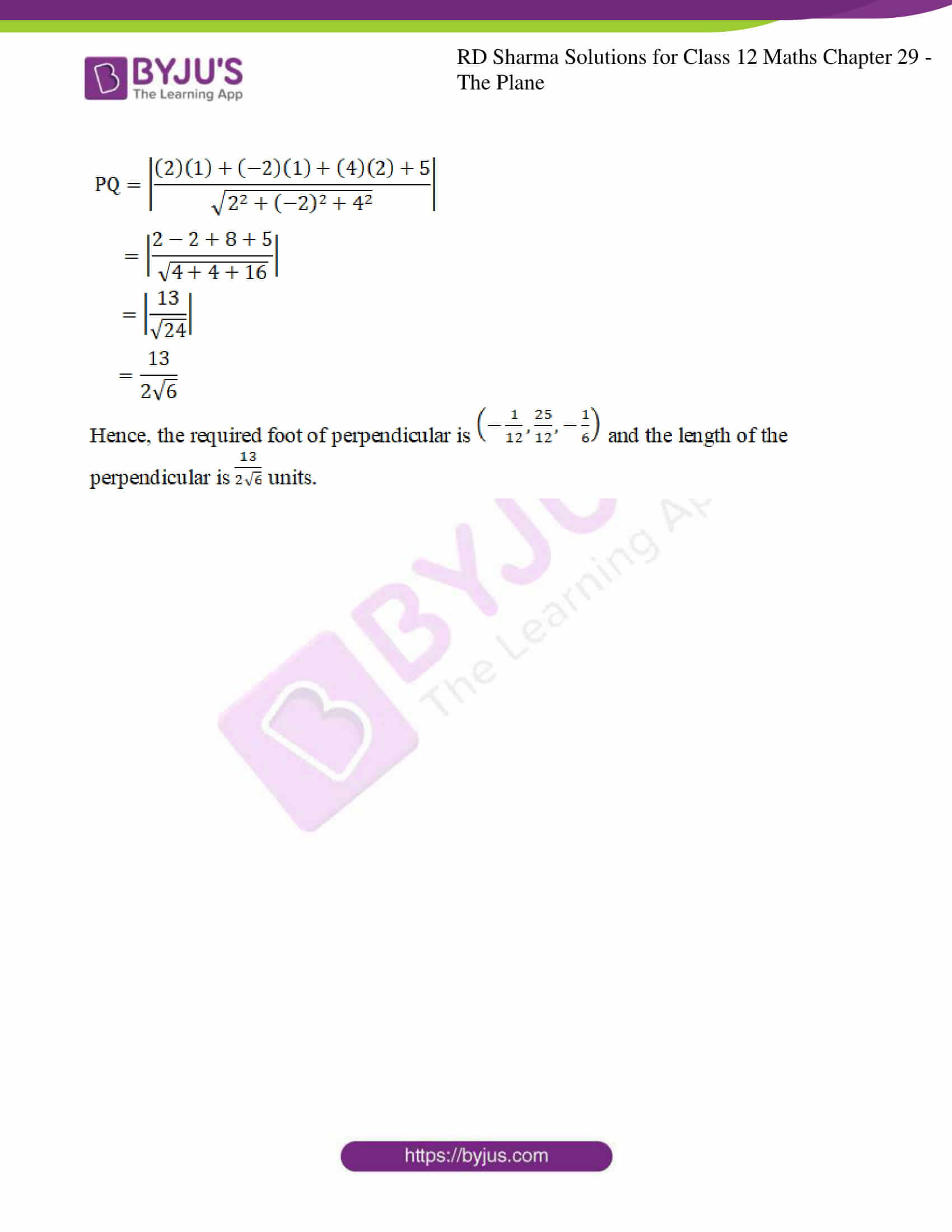### Access RD Sharma Solutions For Class 12 Maths Chapter 29 Exercise 15

EXERCISE 29.15

Q1.

Solution:

Given:

Let point P = (0, 0, 0) and M be the image of P in the plane

3x + 4y – 6z + 1 = 0.

Direction ratios of PM are proportional to 3, 4, – 6 as PM is normal to the plane.

The equation of the line passing through (x1, y1, z1) and having direction ratios proportional to l, m, n is given by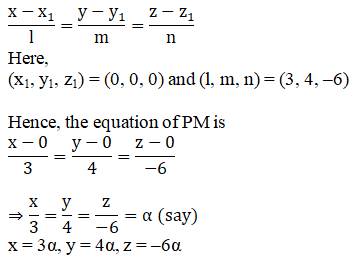Let M = (3α, 4α, –6α).

As M is the image of P in the given plane, the midpoint of PM lies on the plane.

Using the midpoint formula, we have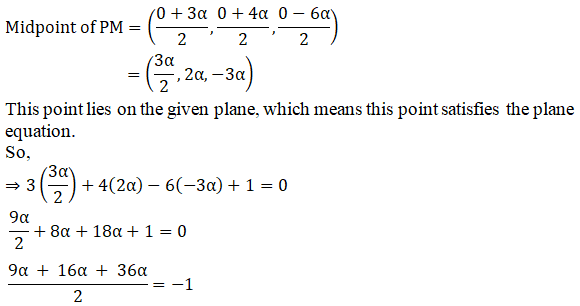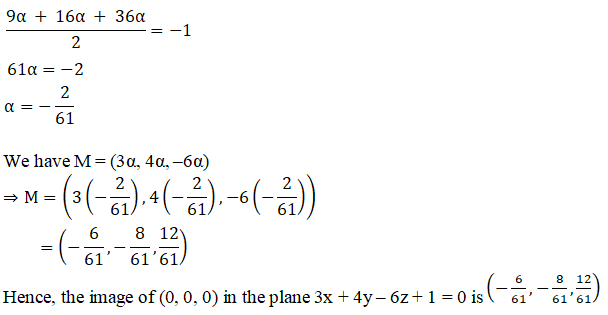Q2.

Solution:

Given:

Let point P = (1, 2, –1) and M be the image of P in the plane 3x – 5y + 4z = 5.

Direction ratios of PM are proportional to 3, –5, 4 as PM is normal to the plane.

The equation of the line passing through (x1, y1, z1) and having direction ratios proportional to l, m, n is given by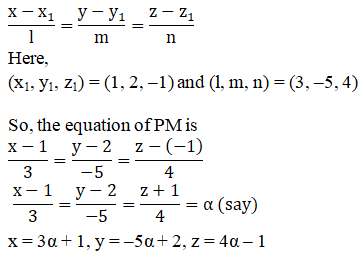Let M = (3α + 1, –5α + 2, 4α – 1).

As M is the image of P in the given plane, the midpoint of PM lies on the plane.

Using the midpoint formula, we have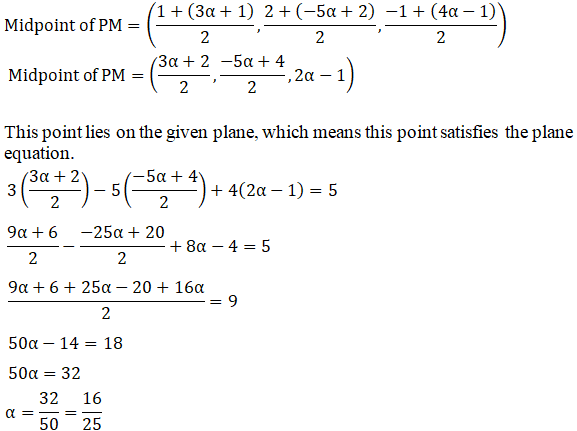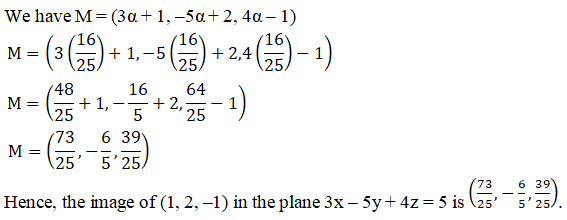Q3.

Solution: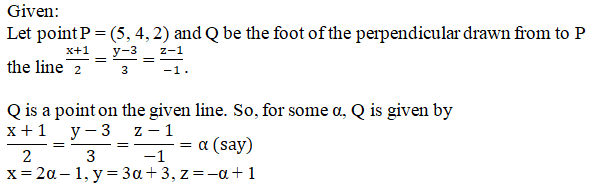So, Q = (2α – 1, 3α + 3, –α + 1)

Now, let us find the direction ratios of PQ.

The direction ratios of a line joining two points (x1, y1, z1) and (x2, y2, z2) are given by (x2 – x1, y2 – y1, z2 – z1).

Here,

(x1, y1, z1) = (5, 4, 2) and (x2, y2, z2) = (2α – 1, 3α + 3, –α + 1)

Direction Ratios of PQ are ((2α – 1) – (5), (3α + 3) – (4), (–α + 1) – (2))

Direction Ratios of PQ are (2α – 6, 3α – 1, –α – 1)

PQ is perpendicular to the given line, whose direction ratios are (2, 3, –1).

We know that if two lines with direction ratios (a1, b1, c1) and (a2, b2, c2) are perpendicular to each other, then a1a2 + b1b2 + c1c2 = 0.

(2)(2α – 6) + (3)(3α – 1) + (–1)(–α – 1) = 0

4α – 12 + 9α – 3 + α + 1 = 0

14α – 14 = 0

14α = 14

α = 1

We have Q = (2α – 1, 3α + 3, –α + 1)

Q = (2×1 – 1, 3×1 + 3, –1 + 1)

Q = (1, 6, 0)

Using the distance formula, we have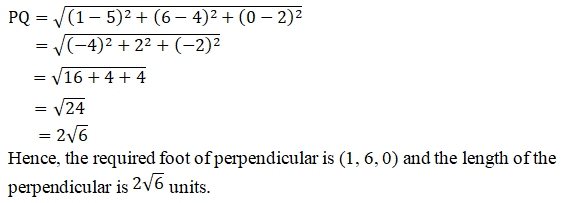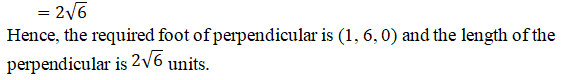Q4.

Solution: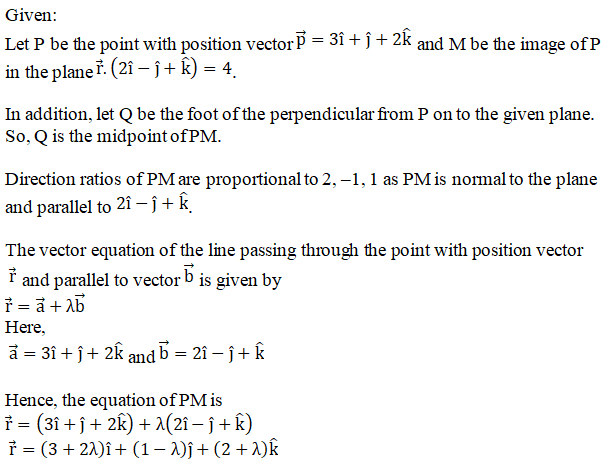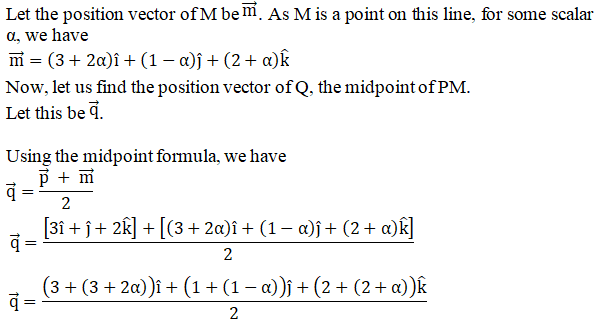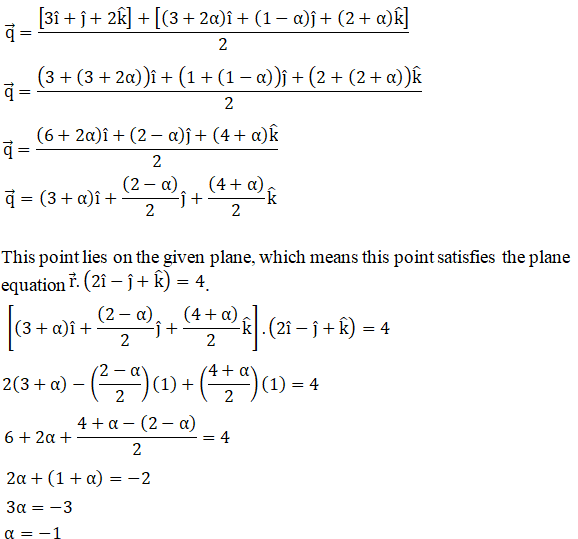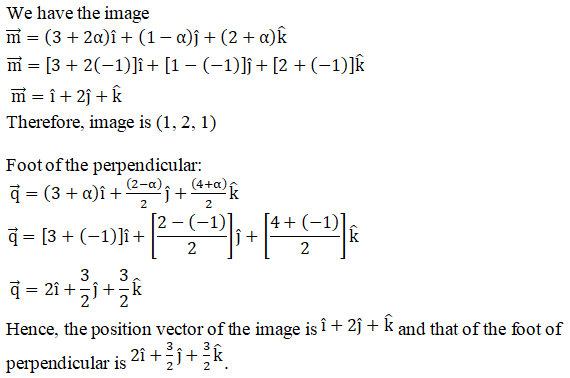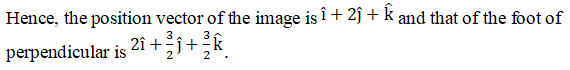Q5.

Solution:

Given:

Let point P = (1, 1, 2) and Q be the foot of the perpendicular drawn from P to the plane 2x – 2y + 4z + 5 = 0.

Direction ratios of PQ are proportional to 2, –2, 4 as PQ is normal to the plane.

The equation of the line passing through (x1, y1, z1) and having direction ratios proportional to l, m, n is given by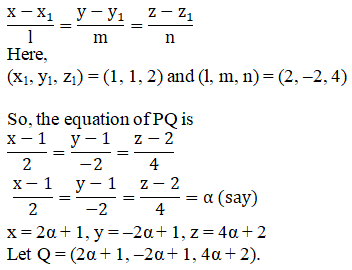This point lies on the given plane, which means this point satisfies the plane equation.

2(2α + 1) – 2(–2α + 1) + 4(4α + 2) + 5 = 0

4α + 2 + 4α – 2 + 16α + 8 + 5 = 0

24α + 13 = 0

24α = –13